# Preface

This section gives an introduction to nonlinear one dimensional oscilaltors. We consider only unforced models leaving driven cases to other sections. Such models turns up in a wide variaty of physical problems and play a major and vital role in the analysis of such systems. Our approach is to demonstrate applications of software in the description of many examples and case studies.

Introduction to Linear Algebra with Mathematica

# Anharmonic motionAn oscillator is a physical system characterized by periodic motion, such as a spring-mass system, which is a classic example of harmonic oscillation when the restoring force is proportional to the displacement. Anharmonic oscillators, however, are characterized by the nonlinear dependence of the restorative force on the displacement. Consequently, the anharmonic oscillator's period of oscillation may depend on its amplitude of oscillation.

There are many systems throughout the physical world that can be modeled as anharmonic oscillators in addition to the nonlinear mass-spring system. In quantum mechanics, an oscillator is described by the Schrödinger equation

${\bf j}\hbar \,\partial_t \,\Psi = H\, \Psi , \qquad \hbar = \frac {h}{2\pi },$
where j is the imaginary unit so j² = -1, ℏ = h/(2 π) is the reduced Planck constant, h ≈ 6.626068 × 10-34 m² kg/s is Planck's constant, an incredibly small number named after the physicist Max Planck who had already guessed this formula in 1900 in his work on black body radiation, and Ψ (the Greek letter psi) is the state vector of the quantum system, t is time, and H is the Hamiltonian operator. The most famous example is the nonrelativistic Schrödinger equation for the wave function in position space of a single particle subject to a potential V, such as that due to an electric field
${\bf j}\hbar \,\frac{\partial}{\partial t} \,\Psi = \left[ \frac{\hbar^2}{2\pi }\, \nabla^2 + V \right] \Psi ,$
where m is the particle's mass, ∇² is the Laplacian, and V is the potential energy of the particle (a function of x, y, z, and t). However, the equation can be separated into temporal and spatial parts (when V is independent of time) using separation of variable $$\Psi (x,t) = \psi (x)\, T(t)$$ to obtain the time-independent Schrödinger equation
$\left[ - \frac{\hbar^2}{2\pi }\, \nabla^2 + V \right] \psi = E\,\psi .$
Here the Hamiltonian operator comes from classical mechanics, which is the sum of the kinetic and potential energies. That is,
$H = \mbox{K} + \Pi .$
In one dimensional case, the Laplace operator becomes just second order derivative operator and we arrive at
$\left[ - \frac{\hbar^2}{2\pi }\, \frac{{\text d}^2}{{\text d} x^2} + V \right] \psi = E\,\psi .$

A usual spring-mass system is governed by Newton's second law:

$m\,\ddot{x} = f(x) ,$
where m is the particle's mass of a weight attached to the spring, x(t) is the displacement from the equilibrium position, and f(t) represents the restoring force. It is useful to introduce "natural units" for length and energy in order the time independent Schrödinger equation and spring-mass equation to be reduced to dimensionless form, which can be written as
$\ddot{x} = f(x) .$
In dissipative systems, the restoring force may depend also on the derivative $$\dot{x} .$$ Since the restoring force can be considered as the derivative of the potential energy, the anharmonic equation in reduced units becomes
$\ddot{x} + \frac{\partial V}{\partial x} =0 ,$
where $$\ddot{x} = {\text d}^2 x/{\text d} t^2$$ is the second derivative with respect to time variable. The potential energy function of a one-dimensional oscillator about its stable equilibrium position, which we take to be x = 0, can usually be expanded in a Taylor series:
$V(x) = V_0 + V_0^{(1)} x + \frac{1}{2!}\,V_0^{(2)} x^2 + \frac{1}{3!}\,V_0^{(3)} x^3 + \frac{1}{4!}\,V_0^{(4)} x^4 + \cdots ,$
where V0 is the value of V(x) at the equilibrium point, and $$V_0^{(n)}$$ is the n-th order derivative of the potential function evaluated at that point. The constant term V0 can be set equal to zero without loss of generality because the potential energy is only defined to within an additive constant. Furthermore, the coefficient of the first order term vanishes since at the equilibrium point, the potential energy function has a local minimum. Consequently, the power series expansion will be
$V(x) = \frac{1}{2!}\,V_0^{(2)} x^2 + \frac{1}{3!}\,V_0^{(3)} x^3 + \frac{1}{4!}\,V_0^{(4)} x^4 + \cdots .$
In order for the potential energy to have local minimum at x = 0, the second order term cannot be negative, $$V_0^{(2)} > 0.$$ Multiplying the differential equation $$\ddot{x} + \frac{\partial V}{\partial x} =0$$ by $$\dot{x} ,$$ we obtain
$\ddot{x} \,\dot{x} + \frac{{\text d}V}{{\text d}x} \,\dot{x} =0 \qquad \Longrightarrow \qquad \frac{{\text d}}{{\text d}t} \left[ \frac{1}{2}\, \dot{x}^2 + V(x) \right] =0 .$
Integration yields
$\frac{1}{2}\, \dot{x}^2 + V(x) = E ,$
the total energy. In many cases, the potential function V(x) can be represented or approximated by a polynomial; however, in some cases it is not the case.

We start by considering one particular anharmonic motion from music, when two real tones are sounded at the same time. In addition to these two tones, one can hear an auxiliary tone or tones that are artificially perceived when two real tones are sounded at the same time. Their discovery is credited to the violinist Giuseppe Tartini (although it was first discovered by the organist George Sorge in about 1745) and so are also called Tartini tones. This effect is most often used in the lowest octave of the organ only. It can vary from highly effective to disappointing depending on several factors, primarily the skill of the organ voicer, and the acoustics of the room the instrument is installed in.

The subjective existence of these combination tones was first investigated theoretically by the German physician and physicist Hermann von Helmholtz (1821--1894). He concluded that the transient displacement x of the tympanic membrane of the ear from its equilibrium position in response to some imposed vibration is governed by the equation

$\ddot{x} + \omega_0^2 x + \alpha\,x^2 =0, \qquad t>0,$
where ω²0 = k/m is the natural frequency of the ear drum and α is a parameter associated with the nonlinear response of the ear drum to the imposed vibration. This equation is often referred to as the anharmonic motion equation as it has subsequently been employed for describing the nonlinear anharmonic oscillations. The initial value problem consisting of the anharmonic equation and usual initial conditions
$x(0) = x_0 , \qquad \dot{x}(0) = v_0 ,$
is now called the Rayleigh problem.

Example: Consider the Rayleigh problem

$\ddot{x} + x + \varepsilon\,x^2 = 0, \qquad x(0) = x_0, \quad \dot{x} (0) = v_0 ,$
that is controlled by the single parameter ε. The corresponding system of differential equations
$\begin{cases} \dot{x} = y , \\ \dot{y} = -x - \varepsilon \, x^2 , \end{cases}$
has two equilibrium solutions: the origin is a center, and $$\left( -1/\varepsilon , 0 \right)$$ is a saddle point (subject to ϵ ≠ 0). We plot phase portraits for different values of ϵ:
Labeled[Grid[{Text@Style[#, "TableHeader"] & /@ {"$Epsilon] = 2", "\[Epsilon] = 1/2" , "\[Epsilon] = 1/6"}, {StreamPlot[{y, -(x + 2*x^2)}, {x, -5, 5}, {y, -5, 5}, StreamColorFunction -> "Rainbow"], StreamPlot[{y, -(x + (1/2)*x^2)}, {x, -5, 5}, {y, -5, 5}, StreamColorFunction -> "Rainbow"], StreamPlot[{y, -(x + (1/6)*x^2)}, {x, -10, 10}, {y, -10, 10}, StreamColorFunction -> Hue] }}, Alignment -> {Center}, Frame -> All, ItemSize -> {{10, 10, 10}}, Spacings -> {0, 1}], Text@ Style[ "Examples of stream plots for different values of parameter \ \[Epsilon]" , "Text"], {{Bottom, Left}}] Stream plot for parameter &epsilon = 2 Stream plot for parameter &epsilon = 1/2 Stream plot for parameter &epsilon = 1/6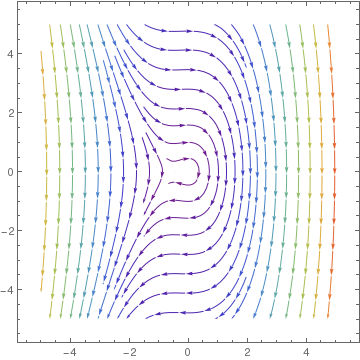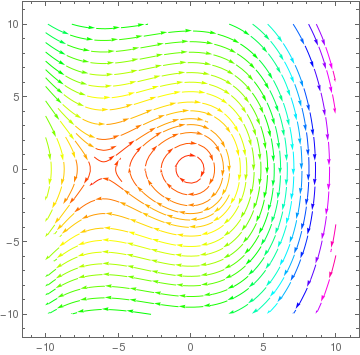To find its energy integral, we multiply the equation by $$\dot{x} = {\text d}x/{\text d}t$$ and integrate with respect to time variable. This yields \[ \frac{1}{2}\, \frac{\text d}{{\text d}t} \left( \dot{x} \right)^2 + \frac{1}{2}\, \frac{\text d}{{\text d}t} \left( x \right)^2 + \frac{\varepsilon}{3}\, \frac{\text d}{{\text d}t} \left( x \right)^3 =0 \qquad \Longrightarrow \qquad \left( \dot{x} \right)^2 + x^2 + \frac{2}{3}\,\varepsilon\,x^3 = C ,$
where
$C = v_0^2 + x_0^2 + \frac{2}{3}\,\varepsilon\,x_0^3 .$
We can also reduce the given second order differential equation to a first order equation by substitution: $$\dot{x} = 1/y .$$ Then
$\ddot{x} = \frac{\text d}{{\text d}t} \left( \dot{x} \right) = \frac{\text d}{{\text d}t} \left( \frac{1}{y} \right) = \frac{\text d}{{\text d}x} \left( \frac{1}{y} \right) \frac{{\text d}x}{{\text d}t} = - \frac{1}{y^2} \, \frac{{\text d}y}{{\text d}x} \, \frac{1}{y} .$
So we get a separable equation
$\frac{{\text d}y}{{\text d}x} = y^3 \left( x^2 + \frac{2}{3}\,\varepsilon\,x^3 \right) .$
Although the above differential equation is a separable one, the corresponding integration leads to a very complicated expression.
StreamPlot[{1, y^3 *(x + 2*x^2)}, {x, -5, 5}, {y, -5, 5}, StreamColorFunction -> "Rainbow"]
StreamPlot[{1, y^3 *(x + (1/3)*x^2)}, {x, -3, 3}, {y, -6, 6}, StreamColorFunction -> Hue]
StreamPlot[{1, y^3 *(x + (1/6)*x^2)}, {x, -10, 10}, {y, -5, 5}, StreamColorFunction -> "Rainbow"]
Stream plot for parameter &epsilon = 2    Stream plot for parameter &epsilon = 1/3    Stream plot for parameter &epsilon = 1/6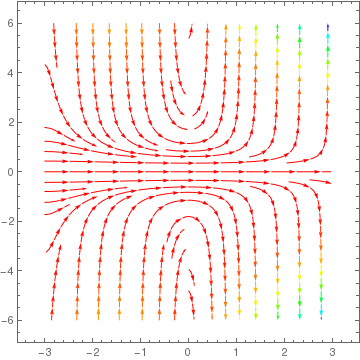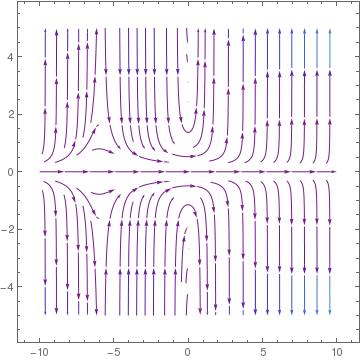There three possibilities.
1. When $$\fbox{\varepsilon > 1/2 }$$   there can be no periodic solution and the solutions must be unbounded.
2. When $$\fbox{\varepsilon = 1/2 }$$   the solution is
$x(t) = 1 - 3\,\tanh^2 \frac{t}{2} ,$
which is a bounded nonperiodic function.
3. When $$\fbox{0 \le \varepsilon < 1/2 }$$   bounded periodic solutions exist.

Example: Consider the mass-spring equation $$\ddot{x} + x - x^2 /2 =0 ,$$ which can be reduced to the following system of first order differential equations

$\left. \begin{array}{l} \dot{x} = y , \\ \dot{y} = -x+ x^2 /2 . \end{array} \right\}$
This system has the two equilibrium solutions: a center at the origin and a saddle point at (2,0). The separatrix is a trajectory that passes through the saddle point.
StreamPlot[{y, (-x + 1/2*x^2)}, {x, -5, 5}, {y, -5, 5}, StreamColorFunction -> "Rainbow"] s = NDSolve[{x'[t] == y[t], y'[t] == -x[t] + (x[t])^2 /2, x == 2, y == 0}, {x, y}, {t, -20, 20}, WorkingPrecision -> 32] ParametricPlot[Evaluate[{x[t], y[t]} /. s ], {t, -20, 20}, PlotRange -> All]Example: We analyze trajectories of hard cubic spring

$\ddot{x} + x + \varepsilon\, x^2 + x^3 /4 =0$
depending on parameter ε. We plot direction fields for ε = 0, 10/9, 200/19, 1, and 3/2.
StreamPlot[{y, -(x + (1)*x^2 + x^3 /4)}, {x, -10, 10}, {y, -10, 10}, StreamColorFunction -> "Rainbow"] LineIntegralConvolutionPlot[{{y, -(x + (10/9)*x^2 + x^3 /4)}, {"noise", 1000, 1000}}, {x, -5.5, 2.5}, {y, -3, 3}, ColorFunction -> "BeachColors", LightingAngle -> 0, LineIntegralConvolutionScale -> 3, Frame -> False]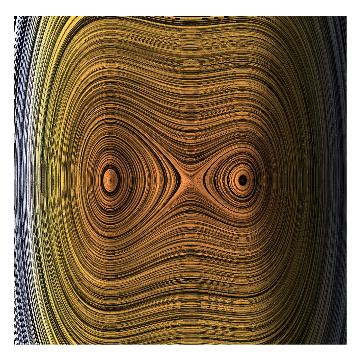Then we use negative ε: -1.5, -1, -200/19, -10/9. ■

Example: Next, we analyze trajectories of soft cubic spring

$\ddot{x} + x + \varepsilon\, x^2 - x^3 /4 =0$
depending on parameter ε. ■

Example:

Show[Graphics[{{Thickness[.05], Line[{{0, 6}, {4, 6}}]}, {Dashed, Line[{{2, 6}, {2, 0}}]}, {Arrowheads[{-.05, .05}],
Arrow[{{1.8, 3}, {1.8, 5.93}}]}, Text[Style["\!$$\*SubscriptBox[\(L$$, $$0$$]\)",
FontSize -> 20], {1.6, 4.5}], {Dashed, Line[{{0, 3}, {4, 3}}]}, Text[Style["x", Italic, FontSize -> 20], {2.35, 2.8}], {Thickness[
0.01], Line[{{2, 6}, {2.2, 5.4}, {2.1, 5.1}, {2.45, 5.2}, {2.15, 4.95}, {2.5, 5.05}, {2.2, 4.8}, {2.55, 4.9}, {2.25,
4.65}, {2.60, 4.75}, {2.3, 4.50}, {2.65, 4.60}, {2.35, 4.35}, {2.70, 4.45}, {2.40, 4.20}, {2.75, 4.3}, {2.45,
4.05}, {2.8, 4.15}, {2.50, 3.9}, {2.85, 4}, {2.75, 3.7}, {3, 3}}]}, Text[Style["L", FontSize -> 20], {2.9, 4.5}],
Disk[{3, 3}, .2], Text[Style["m", FontSize -> 20], {3.35, 3.3}], {Thickness[0.01],
Line[{{3, 3}, {2.75, 2.3}, {2.85, 2}, {2.5, 2.1}, {2.8,
1.85}, {2.45, 1.95}, {2.75, 1.7}, {2.40, 1.8}, {2.70,
1.55}, {2.35, 1.65}, {2.65, 1.40}, {2.3, 1.50}, {2.60,
1.25}, {2.25, 1.35}, {2.55, 1.1}, {2.2, 1.2}, {2.5, .95}, {2.15,
1.05}, {2.45, .8}, {2.1, .9}, {2.2, .65}, {2, 0}}]}, {Thickness[.05], Line[{{0, 0}, {4, 0}}]}}],
ImageSize -> 200]
Consider a particle of mass m, tethered symmetrically by two identical springs that can oscillate along the x axis, as shown in Figure. Each spring is assumed to satisfy Hooke's law with a constant k ans a relaxed for outstretched length ℓ0. The gravitational force is either absent or balanced by some other sources, so that the springs are the only sources of force on the particle. When the particle is at position x, the potential energy of the system is
$V(x) = k \left( \ell - \ell_0 \right)^2 = k\ell_0 \left[ \sqrt{1 + \frac{x^2}{\ell_0^2}} -1 \right] .$

Example:

Show[Graphics[{Line[{{-1.5, 0}, {1.5, 0}}],
Text[Style["x", Italic, FontSize -> 18], {1.7, 0}],
Line[{{0, -1.5}, {0, 1.5}}],
Text[Style["y", Italic, FontSize -> 18], {0, 1.7}], {Opacity[.6],
Disk[{0, .1}, 1, {0, Pi}]}, Disk[{.6, 0}, .07],
Text[Style["m", FontSize -> 18], {.61, -.22}], {Opacity[.6],
Disk[{0, -.1}, 1, {-Pi, 0}]}}], ImageSize -> 300]
Consider a sphere of radius R with a nonuniform, but radially symmetric mass distribution given by the density function $$\rho = \rho_0 (r/R)^s ,$$ where ρ0 is a positive constant and s > -2 (not necessarily an integer).

For a particle of mass m, located at a distance rR from the center of the mass distribution, the gravitational potential energy of the system is given by

$V(r) = \frac{GMm}{(s+2)R} \left( \frac{r}{R} \right)^{s+2} \qquad (r \le R) ,$
where M is the total mass of the sphere, which is related to the other parameters by $$M = (4\pi \rho_0 R^3)/(s+3) .$$ If we choose the x axis so that it passes through the center of the mass distribution with its origin at that point, then along this axis is r = |x|, and
$V(x) = \frac{GMm}{(s+2)R} \left( \frac{|x|}{R} \right)^{s+2} \qquad (|x| \le R) .$
Now suppose we dig a narrow tunnel along the x axis and release the particle from the rest somewhere on the x axis in this tunnel. Simple harmonic oscillations are possible when x = 0, that is, when the mass distribution is uniform (which is not true for the Earth). In this unrealistic case the potential energy reduces to
$V(x) = \frac{GMm}{2R} \, x^2 . (|x| \le R) .$

Example: Consider a particle (or bead) of mass m sliding without friction on a curve (or wire) is a vertical plane (the xy plane), with a minimum at x = 0. The curve can have any profile y = f(x) because a wire can be arbitrary in shape. However, we consider two simple cases when $$y = c\, x^2$$ or $$y = c\, x^4$$ for some positive constant c.

The potential energy will be

$\Pi (s) = mg\, y = mg\,c\, s^2 \qquad \mbox{or} \qquad \Pi (s) = mg\, c\, s^4 .$
Then the total energy becomes
$E = \frac{1}{2}\, m\,\dot{s}^2 + mg\,c\, s^n , \qquad \mbox{where} \quad n = 2,4.$
Differentiating yields
$\ddot{s} + gc\,n\, s^{n-1} =0 \qquad \mbox{for} \quad n=2,4.$
When n = 2, it is just linear equation. For n = 4, we have
$\ddot{s} + 4\,gc\, s^{3} =0 .$
Its phase portrait is shown in the following figure.
StreamPlot[{y, -x^3}, {x, -5, 5}, {y, -3, 3}, StreamScale -> Large]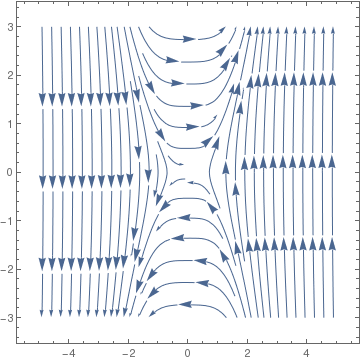Phase portrait for $$\ddot{x} + x^3 =0$$

Example: The Morse potential, named after physicist Philip M. Morse (1903--1985), is a convenient interatomic interaction model for the potential energy of a diatomic molecule. It is a better approximation for the vibrational structure of the molecule than the QHO (quantum harmonic oscillator) because it explicitly includes the effects of bond breaking, such as the existence of unbound states. The celebrated Morse potential (1929) is described by the two-parameter function

$V(x) = V_0 \left[ e^{-2kx} - 2\, e^{-kx} \right] .$
This potential attains minimum at the origin, with a well depth V0 and scale parameter k. For a particle with mass m0, we introduce the dimensionless length and energy variables
$u = k\,x, \qquad \epsilon = \frac{2\,m_0 E}{\hbar^2 k^2}$
as well as a dimensionless potential strength
$K^2 = \frac{2\,m_0 V_0}{\hbar^2 k^2} .$
In these new variables, the Schrödinger equation reads
$\frac{{\text d}^2 \psi}{{\text d} u^2} + \left[ \epsilon - K^2 \left( e^{-2u} - 2\, e^{-u} \right) \right] \psi = 0.$
Its phase portrait is shown in the following figure.
StreamPlot[{y, (Exp[-2*x] - Exp[-x]) - 1}, {x, -3, 5}, {y, -3, 3}, StreamScale -> Large]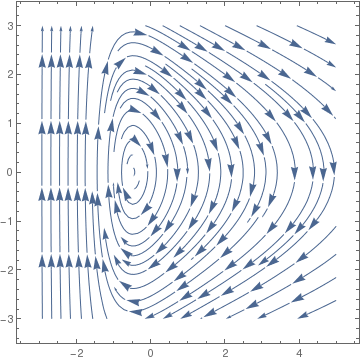Phase portrait for the Morse potential A simple change of variable $$\rho = \sqrt{2K}\, e^{-u/2}$$ transfers the Schrödinger equation into the radial equation of a two-dimensional harmonic oscillator with unit mass and unit angular frequency:
$\frac{1}{\rho}\,\frac{{\text d}}{{\text d}\rho} \left( \rho \, \frac{{\text d}\psi}{{\text d}\rho} \right) + \left[ \frac{4\epsilon}{\rho^2} - \rho^2 + 4\,K \right] \psi = 0 .$

Example: A Pöschl–Teller potential, named after the physicists Herta Pöschl (credited as G. Pöschl) and Edward Teller, is a special class of potentials for which the one-dimensional Schrödinger equation can be solved in terms of special functions. Herta and Edward, while being at Göttingen university, discovered this potential in 1932. After the Nazis came to power in 1933, conditions for Jews in Germany rapidly deteriorated and Teller left the country first to Copenhagen and then to London. In 1935, Teller was recruited at George Washington University, in Washington, D.C. The Pöschl–Teller potential can be defined as

$V(x) = - \frac{V_0}{\cosh^2 (kx)} .$
So the Schrödinger equation with Pöschl–Teller potential becomes
$\frac{{\text d}^2 \psi}{{\text d} x^2} = \left[ \frac{V_0}{\cosh^2 (kx)} - E \right] \psi .$
Upon plotting its phase portrait, we see that it has unstable critical point at (0,0) for small E.
StreamPlot[{y, 1/(Cosh[x])^2 -1}, {x, -5, 5}, {y, -3, 3}, StreamScale -> Large] StreamPlot[{y, 1/(Cosh[x])^2 - 1.2}, {x, -5, 5}, {y, -3, 3}, StreamScale -> Large] StreamPlot[{y, 1/(Cosh[x])^2 - 1/3}, {x, -5, 5}, {y, -3, 3}, StreamScale -> Large]
Stream plot for parameter E = 1.2    Stream plot for parameter E = 1    Stream plot for parameter E = 1/3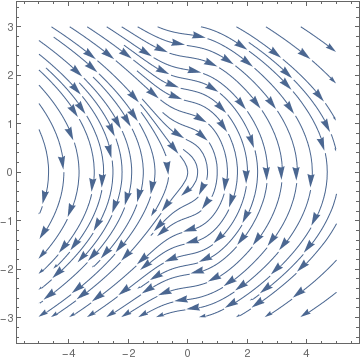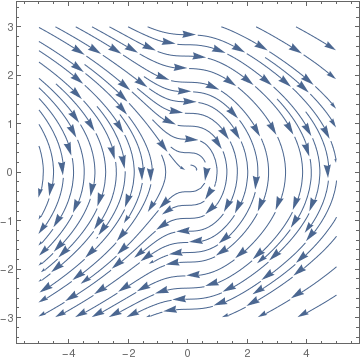Phase portrait for the Schrödinger equation with Pöschl–Teller potential The solutions of this time-independent Schrödinger equation can be found by virtue of the substitution u = tanh(x), which yields the Legendre equation. ■

Example: The trigonometric Rosen–Morse potential, named after the physicists Nathan Rosen and the father of hydrogen bomb Philip M. Morse, is among the exactly solvable quantum mechanical potentials.

$V(x) = - V_0 \left[ \frac{1}{\cosh^2 (kx)} - 2\eta \,\tanh (kx) \right] , \qquad -1 < \eta < 1.$
The Rosen--Morse potential was originally proposed as an analytical model to study the energy levels of the NH3 molecule. ■

Example: The hydrogen atom, consisting of an electron and a proton, is a two-particle system, and the internal motion of two particles around their center of mass is equivalent to the motion of a single particle with a reduced mass. This reduced particle is located at r, where r is the vector specifying the position of the electron relative to the position of the proton. The length of r is the distance between the proton and the electron, and the direction of r and the direction of r is given by the orientation of the vector pointing from the proton to the electron. Since the proton is much more massive than the electron, we will assume that the reduced mass equals the electron mass and the proton is located at the center of mass. The hydrogen atom Hamiltonian also contains a potential energy term, V, to describe the attraction between the proton and the electron. This term is the Coulomb potential energy

$V(r) = - \frac{e^2}{4\pi \epsilon_0 r} ,$
where r is the distance between the electron and the proton. The Coulomb potential energy depends inversely on the distance between the electron and the nucleus and does not depend on any angles. Such a potential is called a central potential. The time-independent Schrödinger equation (in spherical coordinates) for a electron around a positively charged nucleus is then
$\left\{ - \frac{\hbar^2}{2\mu r^2} \left[ \frac{\partial}{\partial r} \left( r^2 \frac{\partial}{\partial r} \right) + \frac{1}{\sin\theta} \,\frac{\partial}{\partial \theta} \left( \sin\theta \,\frac{\partial}{\partial \theta} \right) + \frac{1}{\sin^2 \theta} \, \frac{\partial^2}{\partial \phi^2} \right] - \frac{e^2}{4\pi \epsilon_0 r} \right\} \psi (r, \theta , \phi ) = E\, \psi (r, \theta , \phi ) .$
When the wave function depends on the the radius, we get
$-\frac{\hbar^2}{2\mu} \,\frac{{\text d}^2 u}{{\text d}r^2} - \frac{\hbar^2}{\mu r} \,\frac{{\text d} u}{{\text d}r} - \frac{e^2}{4\pi \epsilon_0 r}\, u = E\, u .$
Using Bohr's radius $$a = \hbar^2 /me^2$$ and the hydrogen ionization energy $${\cal E} = m\.e^4 /2\hbar^2$$ as unit length and unit energy, it is possible to recast the above equation as
$- u'' - \frac{2}{r}\, u' - \frac{2u}{r} = E\,u .$

1. Berrondo, M., Palma, A., Lopez-Bonilla, L., Matrix elements for the Morse potential using ladder operators, International Journal of Quantum Chemistry, 1987, Vol. 26, pp. 243--249.
2. Borghi, R., The variational method in quantum mechanics: an elementary introduction, European Journal of Physics, 2018, Vol. 38, 035410 (16pp).
3. Cevik, D., Gadella, M., Kuru, S., Negro, J., Resonances and antibound states for the Pöschl--Teller potential: Ladder operators and SUSY partners, Physics letters A, 2016, Vol. 380, pp. 1600--1609.
4. Gatland, I.R., Theory of a nonharmonic oscillator, American Journal of Physics, 1991, Vol. 59, No. 2, pp. 155--158; doi: 10.1119/1.16597
5. Kim Johannessen, An anharmonic solution to the equation of motion for the simple pendulum, European Journal of Physics, 2011, Vol. 32, No. 2, pp.407-- doi: 10.1088/0143-0807/32/2/014
6. Mohazzabi, P., Theory and examples of intrinsically nonlinear oscillators, American Journal of Physics, 2004, Vol. 72, No. 4, pp. 492--498; doi: https://doi.org/10.1119/1.1624114
7. Peters, J.M., Bulletin of the Institute of Mathematics and its Applications, 1981, Vol. 17,
8. Usher, J.R. and Nye, V.A., Further observations on the anharmonic motion equation, International Journal of Mathematical Education in Science and Technology, 1989, Vol. 20, No 3, pp. 399--406; doi: 10.1080/00207398902000308